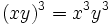# Cube map is surjective endomorphism implies abelian

This article describes an easy-to-prove fact about basic notions in group theory, that is not very well-known or important in itself
View other elementary non-basic facts
VIEW FACTS USING THIS: directly | directly or indirectly, upto two steps | directly or indirectly, upto three steps|

## Statement

### Verbal statement

If the Cube map (?) on a group is an automorphism, or more generally a surjective endomorphism, then the group is an abelian group.

### Statement with symbols

Let$G$ be a group such that the map$\sigma:G \to G$ defined by$\sigma(x) = x^3$ is an automorphism, or more generally, a surjective endomorphism. Then,$G$ is an abelian group.

## Related facts

### Opposite facts

The statement breaks down if we remove the assumption of surjectivity:

Frattini-in-center odd-order p-group implies p-power map is endomorphism: In particular, for$p = 3$, we can obtain non-abelian groups of order$p^3 = 27$, such as prime-cube order group:U(3,3) and semidirect product of Z9 and Z3, where the cube map is an endomorphism. In the former case, the cube map is the trivial endomorphism. In the latter, it is a nontrivial endomorphism.

## Facts used

1. Group acts as automorphisms by conjugation: For any$g \in G$, the map$c_g = x \mapsto gxg^{-1}$ is an automorphism of$G$.
2. nth power map is surjective endomorphism implies (n-1)th power map is endomorphism taking values in the center
3. Square map is endomorphism iff abelian

## Proof

### Hands-on proof using fact (1)

This proof uses a tabular format for presentation. Provide feedback on tabular proof formats in a survey (opens in new window/tab) | Learn more about tabular proof formats|View all pages on facts with proofs in tabular format

Given: A group$G$ such that the map sending$x$ to$x^3$ is a surjective endomorphism of$G$.

To prove:$G$ is abelian.

Proof: We denote by$c_g$ the map$x \mapsto gxg^{-1}$.

Step no. Assertion Facts used Given data used Previous steps used Explanation
1$g^2h^3 = h^3g^2$ for all$g,h \in G$, i.e., every square commutes with every cube Fact (1) Cube map is an endomorphism -- [SHOW MORE]
2$g^2x = xg^2$ for all$g,x \in G$ Cube map is surjective Step (1) [SHOW MORE]
3$g^2x^2 = xgxg$ for all$g,x \in G$ Cube map is an endomorphism -- [SHOW MORE]
4 We get$gx = xg$ for all$g,x \in G$. Steps (2), (3) [SHOW MORE]

### Hands-off proof (using facts (2) and (3))

Given: A group$G$ such that the map sending$x$ to$x^3$ is a surjective endomorphism of$G$.

To prove:$G$ is abelian.

Proof: By fact (2), we conclude that the square map must be an endomorphism of$G$. By fact (3), we conclude that therefore$G$ must be abelian.

### Difference from the corresponding statement for the square map

In the case of the square map, we can in fact prove something much stronger:$(xy)^2 = x^2y^2 \iff xy = yx$

In the case of the cube map, this is no longer true. That is, it may so happen that$(xy)^3 = x^3y^3$ although$xy \ne yx$. Thus, to show that$xy = yx$ we need to not only use that$(xy)^3 = x^3y^3$ but also use that this identity is valid for other elements picked from$G$ (specifically, that it is valid for their cuberoots).